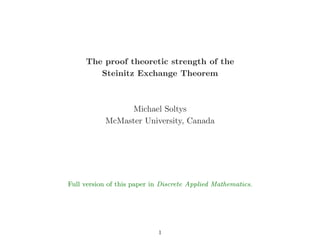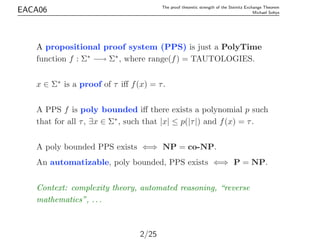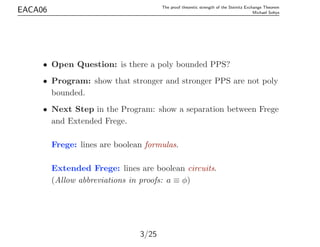Successfully reported this slideshow.

# The proof theoretic strength of the Steinitz exchange theorem - EACA 2006×

1 of 25
1 of 25

# The proof theoretic strength of the Steinitz exchange theorem - EACA 2006

## More Related Content

### The proof theoretic strength of the Steinitz exchange theorem - EACA 2006

1. 1. The proof theoretic strength of the Steinitz Exchange Theorem Michael Soltys McMaster University, Canada Full version of this paper in Discrete Applied Mathematics. 1
2. 2. EACA06 The proof theoretic strength of the Steinitz Exchange Theorem Michael Soltys A propositional proof system (PPS) is just a PolyTime function f : Σ∗ −→ Σ∗ , where range(f) = TAUTOLOGIES. x ∈ Σ∗ is a proof of τ iﬀ f(x) = τ. A PPS f is poly bounded iﬀ there exists a polynomial p such that for all τ, ∃x ∈ Σ∗ , such that |x| ≤ p(|τ|) and f(x) = τ. A poly bounded PPS exists ⇐⇒ NP = co-NP. An automatizable, poly bounded, PPS exists ⇐⇒ P = NP. Context: complexity theory, automated reasoning, “reverse mathematics”, . . . 2/25
3. 3. EACA06 The proof theoretic strength of the Steinitz Exchange Theorem Michael Soltys • Open Question: is there a poly bounded PPS? • Program: show that stronger and stronger PPS are not poly bounded. • Next Step in the Program: show a separation between Frege and Extended Frege. Frege: lines are boolean formulas. Extended Frege: lines are boolean circuits. (Allow abbreviations in proofs: a ≡ φ) 3/25
4. 4. EACA06 The proof theoretic strength of the Steinitz Exchange Theorem Michael Soltys Candidates for the separation: universal principles of matrix algebra, for example: • AB = I → BA = I • pA(A) = 0, pA is the char poly of A, Cayley-Hamilton Thm • det(AB) = det(A) det(B) 4/25
5. 5. EACA06 The proof theoretic strength of the Steinitz Exchange Theorem Michael Soltys Start with the following aim: Show “NC2 concepts” prove the Cayley-Hamilton Thm neg and or 5/25
6. 6. EACA06 The proof theoretic strength of the Steinitz Exchange Theorem Michael Soltys We encode a set of vectors {v1, v2, . . . , vn} as a matrix T = [v1v2 . . . vn]. Steinitz Exchange Theorem (SET): if T is total, and E is linearly independent, then there exists F ⊆ T, such that |F| = |E|, and (T − F) ∪ E is total. ∃X, TX = I ∧ (∀Y = 0, EY = 0) → ∃F ⊆ T, |F| = |E| ∧ ∃X, (T − F ∪ E)X = I So SET is a ΠB 2 formulas of QLA. 6/25
7. 7. EACA06 The proof theoretic strength of the Steinitz Exchange Theorem Michael Soltys Given T, E, the matrix F can be computed in NC2 . Suppose E = [e1e2] and T = [t1t2t3t4], then consider [e1e2] [e1e2t1] [e1e2t1t2] [e1e2t1t2t3] [e1e2t1t2t3t4] Independently for every i = 0, 1, 2, 3, if Rank([e1e2t1 . . . ti]) = Rank([e1e2t1 . . . ti+1]) then put ti+1 in F. Rank can be computed in NC2 with Mulmuley’s algorithm. 7/25
8. 8. EACA06 The proof theoretic strength of the Steinitz Exchange Theorem Michael Soltys Mulmuley’s Algorithma M is n × n matrix, pM (x) its char. poly. (Berkowitz’s alg.) Geometric Rank : usual rank. Algebraic Rank : n − (highest power of x that divides pM (x)). Claim: RankG(M) = RankG(M2 ) ⇒ RankG(M) = RankA(M). Given M, let M be   0 Mt M 0   Clearly, RankG(M) = 1 2 RankG(M ). M = M · diag(1, y, y2 , . . . , y2n−1 ); RankG(M ) = RankG((M )2 ). Here y is an indeterminate, and M is a matrix over the ﬁeld F(y). aParallel Linear Algebra, by Joachim von zur Gathen, chapter in Synthesis of Parallel Algorithms. 8/25
9. 9. EACA06 The proof theoretic strength of the Steinitz Exchange Theorem Michael Soltys Mulmuley’s Algorithma M is n × n matrix, pM (x) its char. poly. (Berkowitz’s alg.) Geometric Rank : usual rank. Algebraic Rank : n − (highest power of x that divides pM (x)). Claim: RankG(M) = RankG(M2 ) ⇒ RankG(M) = RankA(M). Given M, let M be   0 Mt M 0   Clearly, RankG(M) = 1 2 RankG(M ). M = M · diag(1, y, y2 , . . . , y2n−1 ); RankG(M ) = RankG((M )2 ). Here y is an indeterminate, and M is a matrix over the ﬁeld F(y). aParallel Linear Algebra, by Joachim von zur Gathen, chapter in Synthesis of Parallel Algorithms. 9/25
10. 10. EACA06 The proof theoretic strength of the Steinitz Exchange Theorem Michael Soltys Mulmuley’s Algorithma M is n × n matrix, pM (x) its char. poly. (Berkowitz’s alg.) Geometric Rank : usual rank. Algebraic Rank : n − (highest power of x that divides pM (x)). Claim: RankG(M) = RankG(M2 ) ⇒ RankG(M) = RankA(M). Given M, let M be   0 Mt M 0   Clearly, RankG(M) = 1 2 RankG(M ). M = M · diag(1, y, y2 , . . . , y2n−1 ); RankG(M ) = RankG((M )2 ). Here y is an indeterminate, and M is a matrix over the ﬁeld F(y). aParallel Linear Algebra, by Joachim von zur Gathen, chapter in Synthesis of Parallel Algorithms. 10/25
11. 11. EACA06 The proof theoretic strength of the Steinitz Exchange Theorem Michael Soltys Mulmuley’s Algorithma M is n × n matrix, pM (x) its char. poly. (Berkowitz’s alg.) Geometric Rank : usual rank. Algebraic Rank : n − (highest power of x that divides pM (x)). Claim: RankG(M) = RankG(M2 ) ⇒ RankG(M) = RankA(M). Given M, let M be   0 Mt M 0   Clearly, RankG(M) = 1 2 RankG(M ). M = M · diag(1, y, y2 , . . . , y2n−1 ); RankG(M ) = RankG((M )2 ). y is an indeterminate, and M is a matrix over the ﬁeld F(y). aParallel Linear Algebra, by Joachim von zur Gathen, chapter in Synthesis of Parallel Algorithms. 11/25
12. 12. EACA06 The proof theoretic strength of the Steinitz Exchange Theorem Michael Soltys SET can be shown with PolyTime concepts. Can it be shown with NC2 concepts? 12/25
13. 13. EACA06 The proof theoretic strength of the Steinitz Exchange Theorem Michael Soltys SET proves in QLA the following principles 1. (∃B = 0)[AB = I ∨ AB = 0], 2. The columns of an n × (n + 1) matrix are linearly dependent, 3. Every matrix has an annihilating polynomial, 4. AB = I ⊃ BA = I, 5. Existence of An , and the Cayley-Hamilton Thm. 13/25
14. 14. EACA06 The proof theoretic strength of the Steinitz Exchange Theorem Michael Soltys QLA can prove the existence of powers of a matrix from SET. Let POW(A, n) be the formula: ∃ X0X1 . . . Xn (∀i ≤ n)[X0 = I ∧ (i < n ⊃ Xi+1 = Xi ∗ A)] Show QLA (∃B = 0)[AB = I ∨ AB = 0] ⊃ POW(A, n). 14/25
15. 15. EACA06 The proof theoretic strength of the Steinitz Exchange Theorem Michael Soltys Let N be the n2 × n2 matrix consisting of n × n blocks which are all zero except for (n − 1) copies of A above the diagonal zero blocksa . Then Nn = 0, and (I − N)−1 = I + N + N2 + . . . + Nn−1 =         I A A2 . . . An−1 0 I A . . . An−2 ... ... ... 0 0 0 . . . I         . Set C = I − N. Show that if CB = 0, then B = 0, using induction on the rows of B, starting with the bottom row. Using (∃B = 0)[CB = I ∨ CB = 0], conclude that there is a B such that CB = I. aA taxonomy of problems with fast parallel algorithms, by Stephen Cook. 15/25
16. 16. EACA06 The proof theoretic strength of the Steinitz Exchange Theorem Michael Soltys Let N be the n2 × n2 matrix consisting of n × n blocks which are all zero except for (n − 1) copies of A above the diagonal zero blocksa . Then Nn = 0, and (I − N)−1 = I + N + N2 + . . . + Nn−1 =         I A A2 . . . An−1 0 I A . . . An−2 ... ... ... 0 0 0 . . . I         . Set C = I − N. Show that if CB = 0, then B = 0, using induction on the rows of B, starting with the bottom row. Using (∃B = 0)[CB = I ∨ CB = 0], conclude that there is a B such that CB = I. aA taxonomy of problems with fast parallel algorithms, by Stephen Cook. 16/25
17. 17. EACA06 The proof theoretic strength of the Steinitz Exchange Theorem Michael Soltys Let N be the n2 × n2 matrix consisting of n × n blocks which are all zero except for (n − 1) copies of A above the diagonal zero blocksa . Then Nn = 0, and (I − N)−1 = I + N + N2 + . . . + Nn−1 =         I A A2 . . . An−1 0 I A . . . An−2 ... ... ... 0 0 0 . . . I         . Set C = I − N. Show that if CB = 0, then B = 0, using induction on the rows of B, starting with the bottom row. Using (∃B = 0)[CB = I ∨ CB = 0], conclude that there is a B such that CB = I. aA taxonomy of problems with fast parallel algorithms, by Stephen Cook. 17/25
18. 18. EACA06 The proof theoretic strength of the Steinitz Exchange Theorem Michael Soltys Let N be the n2 × n2 matrix consisting of n × n blocks which are all zero except for (n − 1) copies of A above the diagonal zero blocksa . Then Nn = 0, and (I − N)−1 = I + N + N2 + . . . + Nn−1 =         I A A2 . . . An−1 0 I A . . . An−2 ... ... ... 0 0 0 . . . I         . Set C = I − N. Show that if CB = 0, then B = 0, using induction on the rows of B, starting with the bottom row. Using (∃B = 0)[CB = I ∨ CB = 0], conclude that there is a B such that CB = I. Finally, show that B = I + N + N2 + · · · + Nn−1 . aA taxonomy of problems with fast parallel algorithms, by Stephen Cook. 18/25
19. 19. EACA06 The proof theoretic strength of the Steinitz Exchange Theorem Michael Soltys Strong Linear Independence (SLI) if {v1, . . . , vm} are n × 1, non-zero, linearly dependent vectors, then there exists a 1 ≤ k < m such that { lin. indep. v1, . . . , vk, vk+1 lin. dep. , vk+2, . . . , vm} 19/25
20. 20. EACA06 The proof theoretic strength of the Steinitz Exchange Theorem Michael Soltys Csanky’s algorithm (NC2 ) for computing the characteristic poly. of a matrix uses Newton’s symmetric polynomials: s0 = 1, sk = 1 k k i=1 (−1)i−1 sk−itr(Ai ) pA(x) := s0xn − s1xn−1 + s2xn−2 − · · · ± snx0 . 20/25
21. 21. EACA06 The proof theoretic strength of the Steinitz Exchange Theorem Michael Soltys Theorem: QLA proves the Cayley-Hamilton Thm. from SET and SLI. The 12 steps proof : (1) pA is the characteristic polynomial of the matrix A as computed by Csanky’s algorithm. (2) Let W = {ei, Aei, . . . , An ei}. (3) By SET, W must be linearly dependent. (4) By SLI there exists a k ≤ n such that W0 = {ei, Aei, . . . , Ak−1 ei} is linearly independent and k is the largest such index. 21/25
22. 22. EACA06 The proof theoretic strength of the Steinitz Exchange Theorem Michael Soltys (5) Ak ei can be written as a linear combination of the vectors in W0. Let c1, . . . , ck be the coeﬃcients of this linear combination, so that if g(x) = xk + c1xk−1 + · · · + ck, then g(A)ei = 0. (6) Let Ag be the k × k companion matrix of g,           0 0 0 . . . 0 −ck 1 0 0 . . . 0 −ck−1 0 1 0 . . . 0 −ck−2 ... ... ... 0 0 0 . . . 1 −c1           22/25
23. 23. EACA06 The proof theoretic strength of the Steinitz Exchange Theorem Michael Soltys (7) LAP proves pAg = g, and so LAP proves (pAg (A))ei = 0. (8) Extend W0 to B = W0 ∪ {ej1 , . . . , ejn−k }. Existence of B follows from SET: let T = B0 = the standard basis, let E = W0, which is linearly independent, let F = B0 − {ej1 , . . . , ejn−k }, so B = (T − F) ∪ E. A ∼   Ag E1 0 E2   23/25
24. 24. EACA06 The proof theoretic strength of the Steinitz Exchange Theorem Michael Soltys (9) LAP proves that if C1 ∼ C2 then pC1 (x) = pC2 (x) (tr(A) = tr(PAP−1 ), since tr(AB) = tr(BA)). (10) LAP proves that if C =   C1 ∗ 0 C2   then pC(x) = pC1 (x) · pC2 (x). (11) ∴ pA(A)ei = (pAg (A) · pE(A))ei = pE(A) · (pAg (A)ei) = 0. (12) This is true for all ei in the standard basis, and so pA(A) = 0. 24/25
25. 25. EACA06 The proof theoretic strength of the Steinitz Exchange Theorem Michael Soltys Question: Can algorithms be proven correct within the complexity classes that they run in ? 25/25